Courses

# Test: Problems On HCF And LCM- 1

## 10 Questions MCQ Test General Aptitude for GATE 2020 | Test: Problems On HCF And LCM- 1

Description
This mock test of Test: Problems On HCF And LCM- 1 for Quant helps you for every Quant entrance exam. This contains 10 Multiple Choice Questions for Quant Test: Problems On HCF And LCM- 1 (mcq) to study with solutions a complete question bank. The solved questions answers in this Test: Problems On HCF And LCM- 1 quiz give you a good mix of easy questions and tough questions. Quant students definitely take this Test: Problems On HCF And LCM- 1 exercise for a better result in the exam. You can find other Test: Problems On HCF And LCM- 1 extra questions, long questions & short questions for Quant on EduRev as well by searching above.
QUESTION: 1

### Practice Quiz or MCQ (Multiple Choice Questions) with solutions are available for Practice, which would help you prepare for "HCF and LCM" under Quantitative Aptitude. You can practice these practice quizzes as per your speed and improvise the topic. The same topic is covered under various competitive examinations like - CAT, GMAT, Bank PO, SSC and other competitive examinations. Q. Find the greatest number that will divide 43, 91 and 183 so as to leave the same remainder in each case.

Solution:

Given numbers are 43, 91 and 183.
Subtract smallest number from both the highest numbers.
we have three cases: 183 > 43; 183 > 91 and 91 > 43
183 – 43 = 140
183 – 91 = 92 and
91 – 43 = 48
Now, we have three new numbers: 140, 48 and 92.
Find HCF of 140, 48 and 92 using prime factorization method, we get
HCF (140, 48 and 92) = 4
The highest number that divide 183, 91 and 43 and leave the same remainder is 4.

QUESTION: 2

### The H.C.F. of two numbers is 23 and the other two factors of their L.C.M. are 13 and 14. The larger of the two numbers is:

Solution: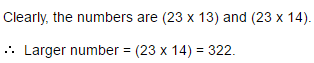QUESTION: 3

### Six bells commence tolling together and toll at intervals of 2, 4, 6, 8 10 and 12 seconds respectively. In 30 minutes, how many times do they toll together ?

Solution: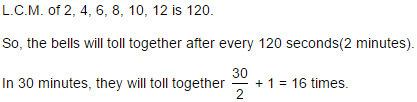QUESTION: 4

Let N be the greatest number that will divide 1305, 4665 and 6905, leaving the same remainder in each case. Then sum of the digits in N is:

Solution:

N = H.C.F. of (4665 - 1305), (6905 - 4665) and (6905 - 1305)

= H.C.F. of 3360, 2240 and 5600 = 1120.

Sum of digits in N = ( 1 + 1 + 2 + 0 ) = 4

QUESTION: 5

The greatest number of four digits which is divisible by 15, 25, 40 and 75 is:

Solution:

Greatest number of 4-digits is 9999.

L.C.M. of 15, 25, 40 and 75 is 600.

On dividing 9999 by 600, the remainder is 399.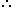Required number (9999 - 399) = 9600.

QUESTION: 6

The product of two numbers is 4107. If the H.C.F. of these numbers is 37, then the greater number is:

Solution:

Let the numbers be 37a and 37b.

Then, 37a x 37b = 4107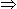ab = 3.

Now, co-primes with product 3 are (1, 3).

So, the required numbers are (37 x 1, 37 x 3) i.e., (37, 111).Greater number = 111.

QUESTION: 7

Three number are in the ratio of 3 : 4 : 5 and their L.C.M. is 2400. Their H.C.F. is:

Solution: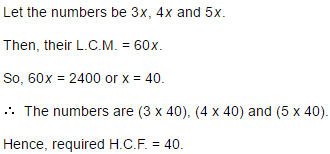QUESTION: 8

The Greatest Common Divisor of 1.08, 0.36 and 0.9 is:

Solution:

Given numbers are 1.08 , 0.36 and 0.90

H.C.F of 108, 36 and 90 is 18                  [ ∵∵ G.C.D is nothing but H.C.F]

Therefore, H.C.F of given numbers = 0.18

QUESTION: 9

The product of two numbers is 2028 and their H.C.F. is 13. The number of such pairs is:

Solution:

Let the numbers 13a and 13b.

Then, 13a x 13b = 2028

⇒ ab = 12.

Now, the co-primes with product 12 are (1, 12) and (3, 4).

[Note: Two integers a and b are said to be coprime or relatively prime if they have no common positive factor other than 1 or, equivalently, if their greatest common divisor is 1 ]

So, the required numbers are (13 x 1, 13 x 12) and (13 x 3, 13 x 4).

Clearly, there are 2 such pairs.

QUESTION: 10

The least multiple of 7, which leaves a remainder of 4, when divided by 6, 9, 15 and 18 is:

Solution:

L.C.M. of 6, 9, 15 and 18 is 90.
Let required number be 90k + 4, which is multiple of 7.
Least value of k for which (90k + 4) is divisible by 7 is k = 4.
∴ Required number = (90 x 4) + 4 = 364.